Monday , May 27 2019
Recent Post
Home / Quantitative Aptitude Tricks / Profit & Loss Shortcut Tips Tricks & Concept

# Profit & Loss Shortcut Tips Tricks & ConceptNABARD Grade A & B Online Course Get Course Now 150+ RRB NTPC Previous Solved Papers PDF Free Download NowRBI Grade B Complete Online Course Register Today RRB JE Free Study Material PDF Books Free Download Now RRB NTPC Free Study Material PDF Free Download NowAll Exam Free Study Group (Free Videos,Pdf,Mock etc) Join Right Now

# Profit & Loss Tips & Tricks

Profit/Loss is another easy topic of Bank & SSC Exams. Most of the questions can be solved in less than 30 seconds. First let me introduce a formula that will be used in solving 50% of the questions.Where,
SP = Selling price
CP = Cost Price
f = Profit/loss factor

What is this profit/loss factor ? It’s simple, ‘f’ depends on the profit/loss %
If profit% = 10, then f = 1.1
If profit% = 30, then f = 1.3
If profit% = 15, then f = 1.15
If loss% = 10, then f = 0.9
If loss% = 25, then f = 0.75
If loss% = 12.5, then f = 0.875
Please note that f depends on profit/loss percentage and not on the absolute value of Profit/Loss. So if in any question it is given that the profit is Rs. 30, then it doesn’t mean that f = 1.3

###Register For NABARD Grade A & B CourseClick Here

####Complete Maths Shortcut eBook > Click To Buy @99rs Only

Let us see some SSC CGL questions that can be solved with this formula

1. 1Let ‘s’ be the SP of 1 article and ‘c’ be the CP of 1 article.
Given, 6c = 4s
Therefore s/c = 1.5
Gain % = 50
Answer : (B)

Q .2.Let ‘s’ be the SP of 1 metre of cloth and ‘c’ be the CP of 1 metre of cloth
Total SP = 20s, Profit = 4s
CP = SP – Profit = 16s
The ratio s/c = 20s/16s = 1.25
Gain % = 25
Answer : (C)

Q . 3.SP of 1 article(s) = Rs. 10/8 = 5/4
CP of 1 article(c) =Rs. 8/10 = 4/5
s/c = 25/16 = 1.5625
Gain % = 56.25
Answer : (A)

1. Q) 4. Kunal sold a shirt at a loss of 10%. Had he sold it for Rs 60 more, he would have gained 5% on it. Find the CP of the shirt.

In this question we have to find the CP of the article

c = s/f
From basic mathematics or elementary science we know that putting delta (∆) sign in numerator and denominator doesn’t change the equation. ∆ stands for ‘change’
c = ∆s/∆f
where ∆s = change in SP
∆f = change in factor
Therefore ∆s = New SP – Old SP = Rs. 60
∆f = New factor – Old factor
New factor is the factor when profit is 5%. Old factor is the one with loss = 10%
So ∆f = 1.05 – 0.9 = 0.15
c = ∆s/∆f = 60/0.15 = Rs. 400
Answer : Rs 4001. 6)CP of 1 article = Rs.8/10 = Rs. 4/5

SP of 1 article = Rs. 10/8 = Rs. 5/4
SP/CP = 25/16 = 1.5625
Hence profit% = 56.25

Answer: (A)

1. 7)Total investment = Total CP
Let the CP of 1 egg = C
Then total CP = 200C

Total eggs left = 200 – 38 = 162 eggs = 162/12 dozen
Total SP = (162/12)*4.8 = Rs. 64.8
f = 1.08
Hence CP = 64.8/1.08 = Rs. 60

Answer: (D)1. 11)This question appeared in Tier-2 2014 and is one of the trickiest question asked by SSC from this topic. So much so that many question banks haven’t published its solution. Although if your concepts are clear, then there is nothing tricky here.

Let the fruit-seller buys 100 oranges

Now, he has sold the oranges in two parts. First he sold 40 oranges and then he sold 48 (80% of 60) oranges.

Total SP = (SP of 40 oranges) + (SP of 48 oranges)

Given, SP of 40 oranges = CP of 100 oranges

40S = 100C (where S = SP of 1 orange and C = CP of 1 orange)

S/C = 2.5

f = 2.5 and hence Profit = 150%

He sold 80% of remaining oranges at half the previous rate of profit.

That means he sold 48 oranges at 75% profit.

CP of 48 oranges = 48C

f = 1.75

SP of 48 oranges = 1.75*48C = 84C

Total SP = (SP of 40 oranges) + (SP of 48 oranges) = 100C + 84C = 184C

Total CP = 100C

f = SP/CP = 184C/100C = 1.84

Hence profit = 84%

Answer: (B)

TRICK-1

If two article are sold at equal prices, the first one is sold at P1% Profit and whereas the second one at a Profit of P2% And the sum of the cost price of two article is x thenFor Example:

(Q1) A trader bought two watches for Rs 2300. He sold one at a profit of 10% and the other at a profit of 20%. If the selling price of each watch is the same, then their cost price are respectively.

Solution: By Trick:C.P of watch at a profit of 10%=((100+20)/(200+10+20))×2300

=(120/230)×2300

=Rs 1200 Ans.

C.P of watch at a profit of 20%=Rs(2300-1200)

=Rs 1100 Ans.

BY CONCEPT:

Let C.P of 1st watch=x

C.P of 2nd watch=2300-x

S.P of 1st watch:x×(100+10)/100=S.P1

11x/10=S.P1

S.P of 2nd watch: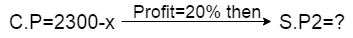(2300-x)×(100+20)/100=S.P2

(2300-x)×120/100=S.P2

(2300-x)×12/10=S.P2

Now According to question: selling price of each watch is the same

SO,

S.P1=S.P2

11x/10=(2300-x)×12/10

11x=2300×12-12x

23x=2300×12

x=Rs 1200 Ans

C.P of 2nd watch=2300-x

=Rs(2300-1200)

=Rs 1100 Ans.

TRICK-2

If two article are sold at equal prices, the first one is sold at a Loss of L1% and whereas the second one at a Loss of L2%, And the sum of the cost price of two article is x thenFor Example:

(Q1) A Man purchases two T.V sets for Rs 21,500. He sold one at a Loss of 12% and the other at a loss of  16%. If the selling price of each T.V is the same, then their cost price are respectively.

Solution: By Trick:C.P of T.V at a Loss of 12%=((100-16)/(200-12-16))×21500

=(84/172)×21500

=Rs 10500 Ans.

C.P of T.V at a Loss of 16%=Rs(21500-10500)

=Rs 11000 Ans.

BY CONCEPT:

Let C.P of 1st T.V =x

C.P of 2nd T.V=21500-x

S.P of 1st T.V: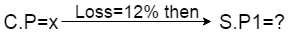x×(100-12)/100=S.P1

88x/100=S.P1

S.P of 2nd T.V:(21500-x)×(100-16)/100=S.P2

(21500-x)×84/100=S.P2

(21500-x)×84/100=S.P2

Now According to question: selling price of each T.V is the same

SO,

S.P1=S.P2

88x/100=(21500-x)×84/100

88x=21500×84-84x

172x=21500×84

x=Rs 1o500 Ans

C.P of 2nd T.V=21500-x

=Rs(21500-10500)

=Rs 11000 Ans.

TRICK-3

If two article are sold at equal prices, the first one is sold at a Profit of P% and whereas the second one at a Loss of L%, And the sum of the cost price of two article is x then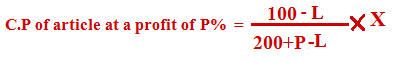For Example:

(Q1) A Man purchases two horses for Rs 16,800. He sold one at a Profit of 25% and the other at a loss of  15%. If the selling price of each horse is the same, then their cost price are respectively.

Solution: By Trick:C.P of horse at a profit of 25%=((100-15)/(200+25-15))×16800

=(85/210)×16800

=(85/210)×16800

=Rs 6800 Ans.

C.P of horse at a Loss of 15%=Rs(16800-6800)

= Rs 10000 Ans.

BY CONCEPT:

Let C.P of 1st horse=x

C.P of 2nd horse=16800-x

S.P of 1st horse: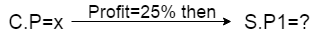x×(100+25)/100=S.P1

125x/100=S.P1

S.P of 2nd horse:(16800-x)×(100-15)/100=S.P2

(16800-x)×85/100=S.P2

(16800-x)×85/100=S.P2

Now According to question: selling price of each horse is the same

SO,

S.P1=S.P2

125x/100=(16800-x)×85/100

125x=16800×85-85x

210x=16800×85

x=Rs 6800 Ans

C.P of 2nd horse=16800-x

=Rs(16800-6800)

=Rs 10000 Ans.

TRICK-4

If two article are sold at equal prices, the first one is sold at a Loss of L% and whereas the second one at a Profit of P%, And the sum of the cost price of two article is x thenFor Example:

(Q1) A trader bought two horses for Rs 19,500. He sold one at a Loss of 20% and the other at a Profit of  15%. If the selling price of each horse is the same, then their cost price are respectively.

Solution: By Trick:C.P of horse at a Loss of 20%=((100+15)/(200-20+15))×19500

=(115/195)×19500

=Rs 11500 Ans.

C.P of horse at a Profit of 15%=Rs(19500-11500)

=Rs 8000 Ans.

(Q1)The total cost price of two watches is Rs 840. One is sold at a profit of 16% and the other at a loss of 12%. There is no loss or gain in the whole transition. Then their cost price is respectively.

Solution: BY CONCEPT

Let C.P of 1st watch=x

C.P of 2nd watch=840-x

S.P of 1st watch: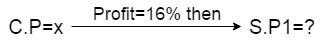x×(100+16)/100=S.P1

116x/100=S.P1

S.P of 2nd watch: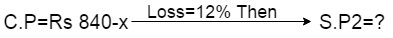(840-x)×(100-12)/100=S.P2

(840-x)×88/100=S.P2

Now According to question: There is no profit or loss

SO,

S.P1+S.P2=840

116x/100+(840-x)×88/100=840

116x+840×88-88x=840×100

28x=84000-73920

28x=10080

∴ x=360 Ans.

C.P of 2nd watch=840-x

=Rs(840-360)

=Rs 480 Ans.

2nd Method: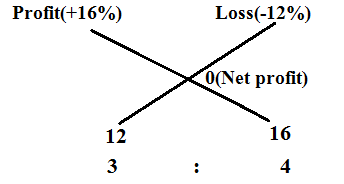C.P of 1st watch at a profit 0f 16%=(3/7)×840

=Rs 360 Ans.

C.P of 2nd watch at a Loss of 12%=(4/7)×840

=Rs 480 Ans

OR

C.P of 2nd watch at a Loss of 12%=Rs(840-360)

=Rs 480 Ans.

(Q2) A man bought a horse and carriage for Rs 40000. He sold the horse at a gain of 10% and the carriage at a loss of 5%. He gained 1% on his whole transaction. The cost price of the horse was.

Solution: BY CONCEPT

Let C.P of horse=x

C.P of a carriage=40000-x

S.P of a horse: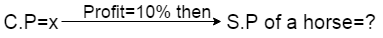x×(100+10)/100=S.P

11x/10=S.P

S.P of a carriage: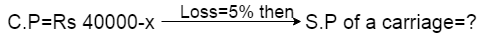(40000-x)×95/100=S.P

Now According to question: he gained 1% on his whole transaction

So,

S.P of a horse+S.P of a carriage=40000×101/100

11x/10+(40000-x)×95/100=40000×101/100

11ox+40000×95-95x=40000×101

15x=40000×101-40000×95

=40000(101-95)

15x=240000

∴ x=Rs 16000 Ans

2nd Method: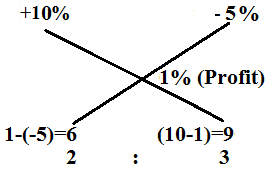C.P of a horse at a profit 0f 10%=(2/5)×40000

=Rs 16000 Ans.

For Example:

(Q1)By selling a chair for Rs 350 instead of Rs 400, loss percent increases by 5%. The cost price of the chair is.

Solution: By Trick:C.P=((400-350)/5)×100

=(50/5)×100

=Rs 1000 Ans.

BY CONCEPT:

S.P1=Rs 350

S.P2=Rs 400

Let C.P=x

Loss1(L1)=x-350

Loss2(L2)=x-400

%L1=((x-350)×100)/x

Note: % loss=(loss×100)/C.P

%L2=((x-400)×100)/x

Now,

L%=L1% – L2%

5=((x-350)×100)/x – ((x-400)×100)/x

5=100/x(x-350 – x-400)

5=(100/x)×50

5x=100×50

x= Rs 1000 Ans.

(Q2)5% more is gained by selling a cow for Rs 1010 than by selling it for Rs 1000. Find the cost price of the cow.

Solution: By Trick: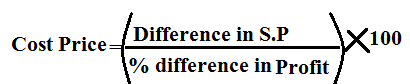C.P=((1010-1000)/5)×100

=(10/5)×100

=Rs 200 Ans.

BY CONCEPT:

S.P1=Rs 1010

S.P2=Rs 1000

Let C.P=x

Profit1(P1)=1010-x

Profit2(P2)=1000-x

%P1=((1010-x)×100)/x

Note: % Profit=(Profit×100)/C.P

%P2=((1000-x)×100)/x

Now,

P%=P1% – P2%

5=((1010-x)×100)/x – ((1000-x)×100)/x

5=100/x(1010-x – 1000-x)

5=(100/x)×10

5x=100×10

x= Rs 200 Ans.

(Q3) A loss of 19% gets converted into a profit of 17% when the selling price is increased by Rs 162. The cost price of the article is.

Solution: By Concept: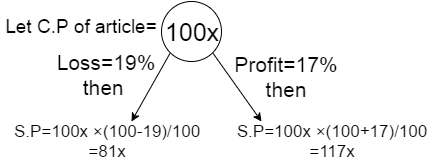[Concept: according to question 19% के loss पर जो selling price है  उसमे Rs 162 increased करने पर loss convert हो गया 17% profit में so 81x+162=117x]

Now,

81x+162=117x

36x=162

∴ x=4.5

C.P=100x=100×4.5

=Rs 450 Ans.

BY TRICK:C.P=(162×100/(17+19)

=(162×100)/36

=Rs 450 Ans.

TRICK:

The profit obtained by selling an article for Rs X is same as the loss incurred if it is sold for Rs Y thenFor Example:

(Q1)The profit obtained by selling an article for Rs 340 is same as the loss incurred if it is sold for Rs 248. Find the C.P.

Solution: By Concept

Let C.P=x

Profit=340-x    [Profit=S.P-C.P]

Loss=x-248      [Loss=C.P-S.P]

Now, According to Question

340-x=x-248

-2x=-588

∴ x= Rs 294

So, C.P=Rs 294 Ans

By Trick:

C.P=(340+248)/2

=Rs 294 Ans

(Q2)The profit obtained by selling a table for Rs 625 is same as the loss incurred if it is sold for Rs 545. The price at which it is to be sold to realize a profit of Rs 65 on the cost price is.

Solution: By Concept

Let C.P=x

Profit=625-x    [Profit=S.P-C.P]

Loss=x-545      [Loss=C.P-S.P]

Now, According to Question

625-x=x-545

2x=1170

x= Rs 585

∴ Required S.P=Rs(585+65)

= Rs 650 Ans

By Trick:

C.P=(625+545)/2

=Rs 585 Ans

∴ Required S.P=Rs(585+65)

= Rs 650 Ans

(Q3)The profit earned by selling an article for Rs 832 is equal to the loss incurred when the same article is sold for Rs 448. What should be the sale price for making 50% profit?

Solution: By Concept

Let C.P=x

Profit=832-x    [Profit=S.P-C.P]

Loss=x-448      [Loss=C.P-S.P]

Now, According to Question

832-x=x-448

2x=1280

x= Rs 640

Now,640×(100+50)/100=S.P

640×(150/100)=S.P

S.P=Rs 960 Ans.

By Trick:

C.P=(832+448)/2

=Rs 640

Now,640×(100+50)/100=S.P

640×(150/100)=S.P

S.P=Rs 960 Ans.

TRICK:

The profit earned by selling an article for Rs X is n times the loss incurred when the same article is sold for Rs Y. Then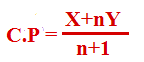For Example:

(Q1)The profit earned by selling an article for Rs 900 is double the loss incurred when the same article is sold for Rs 450. At what price should the article be sold to make 25% profit?

Solution: By Concept

Let C.P=x

Profit=900-x    [Profit=S.P-C.P]

Loss=x-450      [Loss=C.P-S.P]

Now, According to Question

900-x=2(x-450)

3x=1800

x= Rs 600

Now,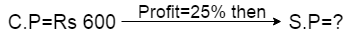600×(100+25)/100=S.P

600×(125/100)=S.P

S.P=Rs 750 Ans.

By Trick:

C.P=(900+2×450)/(2+1)

=1800/3

=Rs 600

Now,600×(100+25)/100=S.P

600×(125/100)=S.P

S.P=Rs 750 Ans.

(Q2)Profit earned by selling an article for Rs 1060 is 20% more than the loss incurred by selling the article for Rs 950. At what price should the article be sold to earn 20% profit?

Solution: By Concept

Let C.P=x

Profit=1060-x    [Profit=S.P-C.P]

Loss=x-950      [Loss=C.P-S.P]

Now, According to Question

1060-x=(120/100)×(x-950)

5(1060-x)=6(x-950)

5300-5x=6x-5700

-11x=-11000

x= Rs 1000

Now,1000×(100+20)/100=S.P

S.P=Rs 1200 Ans.

BY TRICK:

C.P=(1060+(120/100)×950)/(120/100)+1

=(1060+95×12)/(22/10)

=Rs 1000

Now,1000×(100+20)/100=S.P

S.P=Rs 1200 Ans.

(Q3)A shopkeeper sells an article for Rs 140 and earns double the profit that he would have earned had he sold it for Rs 120. What is its cost price?

Solution: By trick

C.P=(2×120-140)/2-1

=Rs 100 Ans.

By Concept:

Let C.P=x

Profit=140-x    [Profit=S.P-C.P]

Profit=120-x

Now, According to Question

140-x=2×(120-x)

x=Rs 100 Ans.

(Q1)A person sells an article at a profit of 10%. If he had bought it at 10% less and sell it for Rs 3 more he would have gained 25%.(1) Find the cost price (2) Find S.P

Solution:Now,

90x×(100+25)/100=110x+3

90x×(125)/100=110x+3

x=6/5

∴ C.P=100x=100×(6/5)

=Rs 120 Ans.

(2)S.P=110x=110×(6/5)

=Rs 132 Ans.

(Q2)A person sold a T.V at a gain of 15 %. Had he bought it for 25% less and sold it for Rs 600 less, he would have made a profit of 32%. The cost price of the T.V was.

Solution: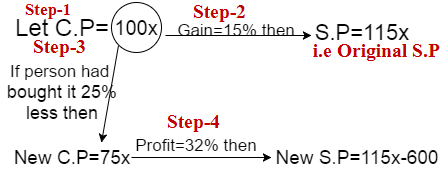Now,

75x×(100+32)/100=115x-600

75x×(132/100)=115x-600

99x=115x-600

x=600/16

C.P=100x=100×(600/16)

=Rs 3750 Ans.

(Q3)A shopkeeper sold a watch at a loss of 20%. But if he could sell it at Rs 200 more, he could earn a profit of 5%. The cost price of the watch is.

Solution: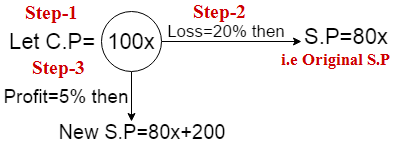Now,

1oox×(100+5)/100=80x+200

105x=80x+200

x=8

∴ C.P of watch=100x=100×8

=Rs 800 Ans

If a trader professes(Profess-दावा करना) to sell his goods at cost price, but uses false weights, then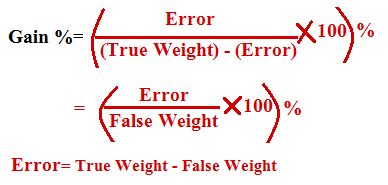For Example:

(Q1) A shopkeeper sells goods at the cost price but uses 900gms instead of 1 Kg. Find his overall profit or loss percentage.

Solution: BY TRICK:

Error= True Weight – False Weight

= 1000g – 900g=100g

% Profit= (100g × 100)/900g

=11 ¹/9% Ans.

BY CONCEPT:

Let C.P of shopkeeper for 1g=Rs 1

C.P of shopkeeper for 1kg ie 1000g=Rs 1000

False C.P of shopkeeper for 900g=Rs 900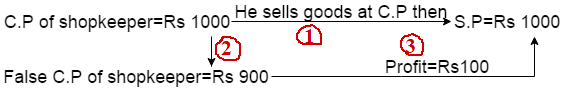[Note: 1,2 and 3 are Steps]

% Profit=(100×100)/900

=11 ¹/9% Ans.

Concept:[दुकानदार ने आप से कहा कि जिस रेट पर मैंने समान(Goods) खरीदा है उसी रेट पर में आप को समान(Goods) दे रहा हूँ| Means दुकानदार ने यहाँ Rs 1000 में समान खरीदा है और आपको भी Rs 1000 में दे रहा है| लेकिन दुकानदार 1 kg समान(Goods) के बदले सिर्फ आपको 900g समान(Goods)दे रहा है| यहाँ समझने वाली बात यह है कि दुकानदार आपसे 1000g(1Kg) का पैसा लिया that means आप ने दुकानदार को Rs 1000 दिया (So S.P of Shopkeeper=Rs 1000) लेकिन उसने सिर्फ आपको 900g समान(Goods) दिया means दुकानदार ने आपको सिर्फ Rs 900 का समान दिया So, C.P of Shopkeeper=Rs 900. ∴ profit=(S.P-C.P)=Rs(1000-900)=Rs 100]

(Q2)A shopkeeper sells his goods at 20% profit and uses 900gms instead of 1 Kg. Find his overall profit or loss percentage.

Solution:

Let C.P of shopkeeper for 1g=Rs 1

C.P of shopkeeper for 1kg ie 1000g=Rs 1000

False C.P of shopkeeper for 900g=Rs 900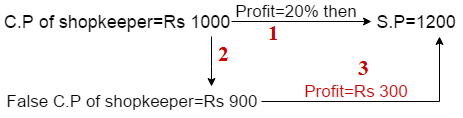% Profit=(300×100)/900

=33 ¹/3% Ans.

Rough:

C.P=Rs 1000 and Profit=20%

S.P=1000×(100+20)/100

=Rs 1200

(Q3) A shopkeeper marks his good at 20% above the cost price and also allows a discount of 20% but uses 800gms instead of 1Kg. Find overall profit or loss percentage.

Solution:

Let C.P of shopkeeper for 1g=Rs 1

C.P of shopkeeper for 1kg ie 1000g=Rs 1000

False C.P of shopkeeper for 800g=Rs 800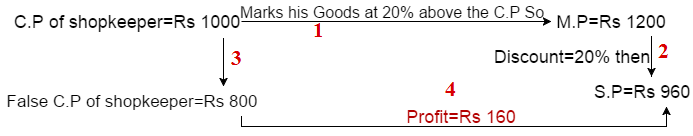% Profit=(160×100)/800

=20% Ans.

Rough:

1000×120/100

=Rs 1200

1200×80/100

=Rs 960

Note: Discount always marked price पर calculate किया जाता है or discount के बाद जो price आता है वह selling price होता है|

(Q4) A dealer sells goods at 6% loss but uses 14g instead of 16g. What is his percentage profit or loss?

Solution:

Let C.P of shopkeeper for 1g=Rs 1

C.P of shopkeeper for 16g=Rs 16

False C.P of shopkeeper for 14g=Rs 14% Profit=(1.04×100)/14

=7.42% Ans.

(Q5) A shopkeeper cheats to the extent of 10% while buying as well as selling, by using false weights. His total gain is.

Solution:=(12100-10000)/100

=21% Ans.

Other Methods: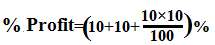=21% Ans.

(Q6)A shopkeeper cheats to the extent of 15% while buying and 20%while selling, by using false weights. His total gain is.

Solution: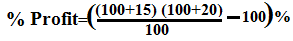=38% Ans.

Other methods:=38% Ans.

For Example:

(Q1)Successive discounts of 30% and 20% are equivalent to a single discount of.

Solution: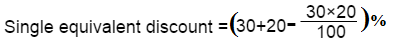=44% Ans.

(Q2)A single discount equivalent to the successive discounts of 10%, 20%, and 25% is.

Solution: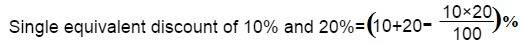=28%

Now,=46% Ans.

(Q3)The difference between successive discounts of 40% followed by 30% and 45% followed by 20% on the marked price of an article is Rs 12. The marked price of the article is.

Solution: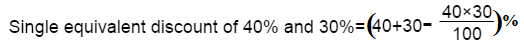=58%=56%

Let M.P=100x

Difference=58%-56%=2%

According to the question

100x×(2/100)=12

so, x=6

∴ M.P=100x=100×6

=Rs 600 Ans.

(Q4)The marked price of an article is Rs 500. It is sold at successive discounts of 20% and 10%. The selling price of the article is.

Solution: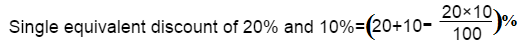=28%

Now,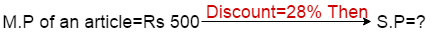500×(100-28)/100=S.P

⇒  500×(72/100)=S.P

∴  S.P=Rs 360 Ans.

(Q5)A trader allows two successive discounts of 30% and 15% on selling an article. If he gets Rs 476 for that article, Find its marked price.

Solution:=40.5%

Now,100x×(100-40.5)/100=476

⇒ 100x×(59.5/100)=476

x=476/59.5

∴ M.P=100x=100×(476/59.5)

=Rs 800 Ans.

(Q6)The difference between a discount of 30% on Rs 2000 and two successive discounts of 25% and 5% on the same amount is.

Solution:

Discount=2000×(30/100)

= Rs 600=28.75%

Now,

Discount=2000×(28.75/100)

= Rs 575

∴ Difference=Rs(600-575)

= Rs 25 Ans.

(Q7)A dealer buys a car listed at 200000 at successive discounts of 10% and 5%. If he sells the car for 179550, then his profit percent is.

Solution:=14.5%

[Concept: suppose dealer company से कार खरीदता है| company car का marked price 2००००० रखता है or company car पर two successive discounts दे रहा है 10% and 5%. इससे company का selling price निकाल लेंगे, company का जो selling price होगा वह dealer का cost price होगा|]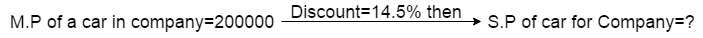200000×(100-14.5)/100=S.P

200000×(85.5/100)=S.P

S.P=Rs 171000

C.P of dealer=Rs 171000

S.P of dealer(Given)=Rs 179550

Gain=Rs(179550-171000)

=Rs 8550

% profit=(8550×100)/171000

=5% Ans

(Q8)A dealer buys a table listed at Rs 1500 and gets successive discounts of 20% and 10%. He spends Rs 20 on transportation and sells it at a profit of 20%. Find the selling price of the table.

Solution: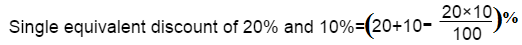=28%

[Concept: suppose dealer company से Table खरीदता है| company Table का marked price 1500 रखता है or company Table पर two successive discounts दे रहा है 20% and 10%. इससे company का selling price निकाल लेंगे, company का जो selling price होगा वह dealer का cost price होगा|]1500×(100-28)/100=S.P

1500×(72/100)=S.P

S.P=Rs 1080

C.P of dealer=Rs 1080

Total C.P of dealer=Rs(1080+20)

=Rs 1100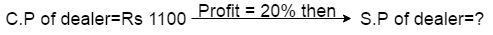1100×(100+20)/100=S.P

1100×(120)/100=S.P

S.P=Rs 1320 Ans.

(Q9)The marked price of a watch is 1600. The shopkeeper gives successive discounts of 10% and x% to the customer. If the customer pays Rs 1224 for the watch. The value of x.

Solution: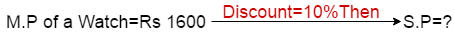1600×(100-10)/100=S.P

⇒1600×(90/100)=S.P

S.P=Rs 1440

Now,

(S.P after x% discount)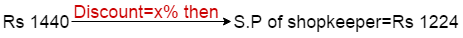[Concept:C.P of customer=1224 i.e S.P of shopkeeper]

1440×(100-x)/100=1224

144000-1440x=122400

x=15% Ans.

(Q10)A bicycle marked at Rs 2000 is sold with two successive discount of 20% and 10%. An additional discount of 5% is offered for cash payment. The selling price of the bicycle at cash payment is.

Solution:=28%

Now,=31.6%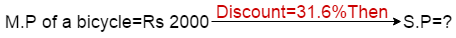=2000×(100-31.6)/100=S.P

=2000×(68.4/100)=S.P

∴ S.P=Rs 1368 Ans.

# Practice Problems On Profit Loss

• A dealer buys a product at Rs. 1920 , he sells at a discount of 20% still he gets the profit of 20% . What is the selling price of that product?
A) Rs.1159
B) Rs.1550
C)Rs.2304
D) Rs.1785
E) Rs.1245
View Answer
Option  C
Solution:
CP ——– SP ———-MP

100—-(20% profit)—-120——-(20% discount) ——150
=> SP = 1.2CP =  2304

• The ratio of cost price and marked price of an article  is 2:3 and ratio of percentage profit and percentage discount is 3:2. What is the discount
percentage ?
A) 18.58%
B) 20.25%
C) 16.66%
D) 22.13%
E) 14.51%
View Answer
Option  C
Solution:
CP : MP = 2x : 3x

=> profit = x
profit % : discount % = 3 : 2
Let CP = 200 , SP = 300
But (3x/100)*200 + (2x/100)*300  = 100
=> x = 8.33%
Discount 2x = 16.66%

• A firm of readymade garments makes both men’s and women’s shirts. Its average profit is 6% of the sales. Its profit in men’s shirts average 8% of the sales and women’s shirts comprise 60% of the output. Find the average profit per sales rupee in women’s shirts .
A) 1.2560
B) 0.0125
C) 0.0566
D) 0.0466
E) 1.1562
View Answer
Option D
Solution:
According to questions,

women’s shirts comprise 60% of the output.
Therefore, Men’s shirts comprise 40% of the output.
Average profit from men’s shirts = 8% of 40 = 3.2 out of 40
Overall average profit = 6 out of 100
Average profit from women’s shirts = 2.8 out of 60
= 0.0466 out of each shirt.

• A shopkeeper marks his goods 20%  above his cost price and gives 15% discount on the marked price. Find his gain%.
A) 2%
B) 8%
C) 11%
D) 6%
E) 7%
View Answer
Option
Solution:
CP = 100 , MP = 120

D = (15/100)*120 = 18%
SP = 102
P% = (P/CP)*100 = (2/100)*100 = 2%

• A, B and C invest in the ratio of 3 : 4 :5 . The percentage of return on their investments are in the ratio of 6 : 5 : 4 . Find the total earnings, if B earns Rs. 250 more than A.
A) 7500
B) 6999
C) 4575
D) 7250
E) 2500
View Answer
Option D
Solution:
A ————– B ————– C

Invetsment          3x ———— 4x ————— 5x
Rate of return     6y% ———-5y%————— 4y%
Return                (18xy/100)—-(20xy/100)—–(20xy/100)
Total = (18+20+20) = 58xy/100
B’s earnings  – A’s earnings = 2xy/100 = 250
Total earnings = 58xy/100 = 7250

• Jagran group launched a new magazine in January 2004. The group printed 10000 copies initially for Rs. 50000. It distributed 20% of its stock freely as specimen copy and 25% of the rest magazines are sold at 25% discount and rest at 16.66% discount whose printing price was Rs. 12 per copy . What is the overall gain or loss in the first month’s issue of magazine, if the magazine could not realize the income from advertisements or other resources?
A) 56%
B) 62%
C) 74%
D) 50%
E) 68%
View Answer
Option A
Solution:
Total cost = Rs.50,000

Total sale price  = 2000 * 9 + 6000 * 10 = 78,000
Profit% = (28000/50000)*100 = 56%

• A dishonest trader marks up his goods by 80% and gives discount of 25% . Besides he gets 20% more amount per kg from wholeseller and sells 10% less per kg to customer. What is the overall profit percentage ?
A) 63%
B) 72%
C) 88%
D) 55%
E) 80%
View Answer
Option E
Solution:
CP = 100/120 = 10/12

SP = 135/90 = 18/12
Profit % = {[(18/12) – (10/12)]/(10/12)} *100 = 80%

• By selling 12 marbles for a rupee, a shopkeeper loses 20% . In order to gain 20% in the transaction , he should sell the marbles at the rate of how many  marbles for a rupee?
A) 14
B) 8
C)11
D) 9
E) 22
View Answer
Option B
Solution:
SP of 12 marbles  = Rs.1 , loss = 20%

CP of 12 marbles = Rs. (1/0.8) = Rs. 1.25
SP of 12  marbles at a gain of 20%
CP * 1.2 = 1.25 *1.2
= Rs. 1.5
It means in order to gain 20%, he should sell 12 marbles for Rs.1.5
For Rs. 1 , he should sell 12/1.5 = 8 marbles

• A shopkeeper bought a DVD marked at Rs. 200 at successive discounts of 10% and 15% respectively. He spent Rs. 7 on transport and sold the table for Rs.208 .What will be his profit percentage ?
A) 58%
B) 44%
C) 30%
D) 50%
E) 62%
View Answer
Option C
Solution:
Single equivalent discount for 10% and 15% = (15 + 10  – (15*10/100))%

= 23.5%
CP of DVD = 200*(100- 23.5)% = Rs.153
Expense on transport = Rs. 7
Actual CP = 153 + 7 = Rs. 160
Profit % = [(208 – 160)/160]*100 = 30%

• The cost of setting up a magazine is Rs.2800. The cost of paper and ink is Rs.80/ 100 copies and printing  cost is Rs. 160 / 100 copies. In the last month 2000 copies were printed but only 1500 copies could be sold at Rs. 5 each . Total 25% profit on the sale price was realized. There is one more resource of income from the magazine which is advertising. What sum of money was obtained from the advertising in magazine?
A) Rs.1654
B) Rs.1522
C) Rs.1750
D) Rs.1975
E) Rs.1800
View Answer
Option D
Solution:
Setup cost = Rs.2800

Paper , ink = Rs. 1600
Printing cost = Rs. 3200
Total cost = Rs. 7600
Total sale price = 1500*5 = 7500
Let the amount obtained from advertising is x,
then
(7500+x) – 7600 = 25% of 7500
=> x = 1975

• A dealer offers a discount of 20% and still makes a profit of 20% and he further allows 4 articles free on the sale of 12 articles. Find the ratio of cost price to market price.
A) 1:2
B) 4:5
C) 3:7
D) 2:5
E) 5:7
View Answer
Option A
Solution:
Formula : MP(1 – d%) = CP(1+g%)

MP(80/100) = CP(120/100)
CP/MP = 80/120———(1)
Now,
16 articles given in the cost of 12 articles
MP of one article = total /12——–(2)
CP of one article = total /16———(3)
For one article:
CP/MP= (80/16)/(120/12) = 1/2

• A and B are dealers of a bike company. The price of a bike is Rs.28,000. A gives a discount of 10% on whole , while B gives a discount of 12% on the first Rs.20,000 and 8% on the rest Rs.8000. What is the difference between their selling price?
A) Rs.110
B) Rs.180
C) Rs.240
D) Rs.200
E) Rs.90
View Answer
Option C
Solution:
A’ s discount = 2800

B’s discount = 2400+640 = 3040
Required difference = 3040-2800 = Rs.240

• Sonata sells a wrist watch to a wholeseller making a profit of 10%. The wholesaler sells it to the retailer making a profit of 10%. A customer purchases it by paying Rs.990. Thus the profit of retailer is 2(3/11)% what is the cost incurred by the Sonata to produce it?
A) Rs.755
B) Rs.950
C)Rs.850
D) Rs.550
E) Rs.800
View Answer
Option E
Solution:
[((x*1.1)*1.1)*(1125/1100)] = 990

=> x = 800

• Fanta and Coke, there are two companies, selling the packs of cold-drinks. For the same selling price Fanta gives two successive discounts of 10% and 25%. While Coke sells it by giving two successive discounts of 15% and 20%. What is the ratio of their marked price?
A) 110:111
B) 120:125
C) 131:133
D) 136:135
E) 140:141
View Answer
Option D
Solution:
Fanta *0.9*0.75 = Coke *0.85*0.80

Fanta/Coke = 136/135

• Profit on selling 10 candles equals selling price of 3 bulbs. While loss on selling 10 bulbs equals selling price of 4 candles. Also profit percentage equals to the loss percentage and cost of a candle is half of the cost of a bulb. What is the ratio of selling price of candle to the selling price of a bulb?
A) 2:1
B) 3:2
C)7:9
D) 5:3
E) 3:1
View Answer
Option B
Solution:
Candle  —————— Bulb

CP ….x ——————– y
SP…..a———————b
and   y = 2x
Profit  = 10(a – x) = 3b
Loss     = 10(y – b) = 4a
Profit% = (3b/10x)*100———–(1)
and Loss% = (4a/10y)*100———-(2)
Again,
equating (1) & (2), we get
a/b = 3/2

• A person wants to reduce the trade tax so he calculates his profit on the sale price instead of on the cost price. In this way by selling a article for Rs. 280 he calculates his profit as 14(2/7)%. What is his actual profit percentage ?
A) 20.12%
B) 16.66%
C) 15.66%
D) 22.21%
E) 31.11%
View Answer
Option B
Solution:
CP ————-SP

240…..(- 14 2/7)……280
Actual profit% = (40/240)*100 = 16.66%

• A  person sold an electronic watch at Rs. 96 in such a way that his percentage profit is same as the cost price of the watch . If he sells it at twice the percentage profit of its previous percentage then find the new selling price.
A) Rs.132
B) Rs.120
C) Rs.123
D) Rs.100
E) Rs.110
View Answer
Option A
Solution:
SP = x + (x*x)/100 = 96%

=> x = 60
New , SP = 60+(60*120)/100 = Rs. 132

• A bookseller procures 40 books for Rs. 3200 and sells them at a profit equal to the selling price of 8 books. What is the selling price of one dozen books, if the price of each book is same?
A) Rs.1300
B) Rs.1100
C) Rs.800
D) Rs.1200
E) Rs.1000
View Answer
Option D
Solution:
CP = Rs. 3200/40 = Rs.80

Now SP of 40 books =  CP of 40 books + SP of 8 books
=> SP of 32 books = 3200
SP of 1 book = Rs.100
Therefore,
Required SP of 1 dozen books  = Rs. 1200

• A firm of readymade garments makes both men’sand women’s shirts. Its average profit is 6% of the sales. Its profit in men’s shirts average 8% of the sales and women’s shirts comprise 60% of the output. What is the average profit per sales rupee in women’s shirts.
A) 0.1243
B) 0.5416
C) 0.0466
D) 0.5247
E) 0.2451
View Answer
Option C
Solution:
Women’s shirts comprise = 60%

Men’s shirts comprise =40%
Average profit from men’s shirts = 8% of 40 = 3.2 out of 40
Overall average profit = 6 out of 100
Average profit from women’s shirts = 2.8 out of 60 i.e. 0.0466 out of each shirt.

• Of the two varieties of rice available, variety A is bought at Rs.32 per kg and variety B at Rs.80 per kg. Two varieties of rice are mixed together in the respective ratio of 8:5 and the mixture is sold at Rs.72 per kg. What per cent of profit approximately the seller receives ?
A) 50%
B) 40%
C) 30%
D) 55%
E) 43%
View Answer
Option E
Solution:
Let 8 kg of first variety of rice and 5 kg of second variety is mixed.

CP of 13 kg of rice = (8*32 + 5*32) = Rs. 656
SP of 13 kg of rice = 72*13 = Rs.936
Profit = 936 – 656 = Rs.280
Profit% = (280/656)*100 = 43%

1. Raman calculates his profit % on the selling price whereas Rajeev calculates his on the cost price. They find that the difference of their profits Rs.150. If the selling price of both the m are the same and both of them get 50% profit. Find their selling price.
A) Rs. 620
B) Rs. 900
C) Rs.870
D) Rs.750
E) Rs.550
View Answer
Option B
Solution:
selling price = [Diff. * 100 * (100 + 50)]/(50)^2

=  [150 * 100 * 150 ]/ 2500 = 900

2. A trader has 600kgs of rice ,  a part of which he sells at 15% profit and the remaining quantity at 20% loss. On the whole , he incurs an overall loss of 6% .What is the quantity of rice he sold at 20% loss?
A) 300kg
B) 410kg
C) 360kg
D) 210kg
E) 500kg
View Answer
Option C
Solution:
Quantity  of rice sold at 20% loss = x kg (let)

Quantity of rice sold at 15% gain  = (600 – x ) kg
(600 – x) * (115/100) + (x*80)/100 = (600 * 94)/100
=> x = 12600/35 = 360 kg

3. A camel and a cart together cost Rs. 5000.If  by selling the camel at a profit of 10% and the cart at a loss of 10% a total profit of 2.5% is made ,then what is the cost price of the camel ?
A) Rs.4500
B) Rs. 3125
C) Rs. 3000
D) Rs.2100
E) Rs.3800
View Answer
Option B
Solution:
Let the cp of the camel and the cart be x and (8000-x) resp.

sp of camel = 1.1x
sp of cart = 0.9(5000-x)
Therefore ,
1.1x * 0.9(5000 – x) = 5000 * 1.025
=> x = Rs. 3125

4. If there is a loss of  40% when a good is sold at (2/5)th of its earlier selling price. Find the profit%  after selling the good at a certain price.
A) 24.15%
B) 31.3%
C) 47%
D 35%
E) 50%
View Answer
Option E
Solution:
CP = (2/3) of SP

SP = (100+P) / 100 of CP
Equating both the above equations,
Profit = 50%

5. There are two watches of cost Rs. 800. One is sold at a profit of 16% and the other at a loss of 8%. If there is no loss  or no gain in the whole transaction, the cost price of the watch on which the shopkeeper gains is?
A) Rs. 400
B) Rs.740
C) Rs.504
D) Rs.450
E) Rs.645
View Answer
Option  A
Solution:
profit …………….loss

+16 …………….. -8
———–0
8……………16
1 : 2
Therefore ,
price of watch sold at profit = (1/2) * 800 =  Rs. 400
price of watch sold at loss = 2 * 800 = Rs. 1600

6. Niel bought 30kg rice at the rate of Rs.9.50/kg and 40kg of rice at the rate of Rs. 8.50/kg and mixed them. She told the mixture at the rate of Rs. 8.90/kg. Find the total profit or loss in the whole transaction.
A) Rs.4 profit
B) Rs.2 loss
C) Rs.2 profit
D) Rs.4 loss
E) None of these
View Answer
Option B
Solution:
Total CP = 30 *9.50 + 40 * 8.50 =625

Total SP = 8.9(30+40) =623
Loss = Rs.2

7. If a shopkeeper sell a TV at 15% profit and a DVD at 12% loss then he earns Rs. 540 as total profit but if he sells the TV at 12% loss and the DVD at 15% proft then there is no loss or profit.Find the price of the TV and the DVD ?
A) Rs.11,000 and Rs. 2000
B) Rs.15,000 and Rs. 3000
C) Rs.11,000 and Rs. 6000
D) Rs.10,000 and Rs.8000
E) Rs.17,000 and Rs. 4000
View Answer
Option D
Solution:
.             TV ……………… DVD

CP—–500…………….400
SP—–+15%…………   -12%
P = 75……………  L = -48
Total profit  = 75 – 48 = Rs. 27
27 *20 = Rs. 540
CP of TV = 500 * 20 = Rs . 10,000
CP of DVD = 400 * 20 = Rs. 8000

8. A cloth merchant uses 120cm scale while buying,instead a meter scale ,but uses an 80 cm scale while selling the same cloth. If he offers a discount of 20% on cash payment. Find the profit percent.
A) 40%
B) 35%
C) 20%
D) 15%
E) 30%
View Answer
Option C
Solution:
After discount = (80 * 120)/100 = 96

Profit = 96 – 80 = 16
Therefore , Profit% = (16*100)/80 = 20%

9. An article is marked 50% over its cost price.Two successive discounts of 12% and 13(1/2)% are allowed on the marked price of the article.Find the profit or loss percent after selling at discount.
A) 8%
B) 10%
C) 13%
D) 14.18%
E) 7.4%
View Answer
Option D
Solution:
Let the CP be Rs. 100

SP = 150 * (88/100) * (86.5/100) = Rs. 114.18
Therefore ,
= (14.18/100)*100 =14.18%

10. A trader marked his goods at such a price that after allowing a discount of 12(1/2) % for cash payment, he makes a profit of 20% . What is the marked price of the good which costs Rs. 210 ?
A) Rs.300
B) Rs.210
C) Rs.470
D) Rs. 288
E) Rs.200
View Answer
Option D
Solution:
Required MP = [210 * (100+20)]/(100-12.5) = (210 *120)/87.5 = Rs. 288

• If a person reduce his selling price of an article by rs40 then the suggested profit of 33(1/3)% convert into loss of 20%. Find the cost price?
A) Rs60
B) Rs75
C) Rs100
D) Rs150
View Answer
Option B
Solution:

.                                        C.P………………S.P
33(1/3)% gain=1/3            3………………….4
20%LOSS= 1/5                 5………………….4
Make C.P same & we will get
15………..20
15…………12
20-12 =8
8 =40
1 =5
15 =75

• The price of an article increase by 20% and a man now get 10kg less, if he also reduce his consumption by 20%, then find how much kg of article he used to purchase in normal price?
A) 15kg
B) 20kg
C) 30kg
D) 40kg
View Answer
Option C
Solution:

.       100
80             120
120 -80 =40
40/120 * T =10
T = 30kg

• A shopkeeper sell his goods at 25% loss but he uses false weight of 30%. Find the loss or profit of shopkeeper in this whole process?
A) 50/7% profit
B) 50/3% profit
C) 50/7% loss
D) 50/3% loss
View Answer
Option A
Solution:

.          1000
700                 750
750 – 700 = 50
50/700 *100 = 50/7% profit

• A shopkeeper sells an article at 25% profit. Had he bought it for 10% loss and sold it for rs16 less, he would have earned 30% profit. What is the actual cost price?
A) 100
B) 150
C) 175
D) 200
View Answer
Option D
Solution:

let C.P = 100
CP………………….SP
100……………….125
At 10% loss
90………………….117…..125- 117= 8
.         +30% profit
8 =16
1 =2
100 =200

• A dishonest milkman sells milk at cost price but he mixes water and earns 14(2/7)%. Find the ratio of mixture and milk in the mixture?
A) 7 : 1
B) 7 : 8
C) 1 : 7
D) 8 : 7
View Answer
Option D
Solution:

milk : water
100 14(2/7)
7 : 1
Milk =7
Mixture = 7+1 =8
Mixture : milk
8 : 7

• A man sells his two articles at Rs1200. At one he gains 25% and at another he gains 16(2/3)%. Overall he gains 20%. Find the cost price of first article?
A) 500
B) 400
C) 600
D) 700
View Answer
Option B
Solution:

first we find C.P of both
20% gain = 1/5
SP is 1200, so 1+5 = 6 = 1200. SO 5 = 1000
25……………………………….50/3
…..                 20
10/3…………………………..5
2 : 3
2/5*1000 = 400

• A sold an article to B at the profit of 25%, B sold it to C at loss of 10% and C sold it to D at the profit of 20%. If D paid rs27, then how much A paid to buy this article?
A) Rs20
B) Rs15
C) Rs12
D) Rs9
View Answer
Option A
Solution:

125/100 * 90/100 * 120/100 * X = 27
X = rs20

• A man buys some toffees at 5 toffee in 3rupee and same number of toffee at 5 toffee in rs4. He sold all of them at 5 toffee in 4 rupee. Find his overall gain or loss percent?
A) 16(2/3)%
B) 10%
C) 20%
D) 14(2/7)%
View Answer
Option D
Solution:

.           Rs………..Qty
.             3………….5
.             4………….5
C.P       Rs7      10toffees
He sold at 5toffees in Rs4 so for 10toffees he gets Rs8
C.P =Rs27………. S.P = Rs28
Profit % = 1/7 *100 = 14(2/7) %

• By selling 144 hens Mahesh suffer a loss equal to the selling price of 6 hens. Find his loss percent?
A) 4%
B) 3%
C) 9%
D) 4(1/2)%
View Answer
Option A
Solution:

loss = C.P – S.P
S.P of 6 hen = C.P of 144 hens – S.P of 144 hens
S.P of 150 hens = C.P of 144 hens
6/150 *100 = 4% loss

• If a man wants to gain 33(1/3)% after allowing a discount of 16(2/3)%. Then find how much percent he has to increase his C.P to make M.P ?
A) 33(1/3)%
B) 50%
C) 60%
D) 66(2/3)%
View Answer
Option C
Solution:

gain 33(1/3)% =1/3
C.P …………………..S.P
3…………………………4
Discount 16(2/3)% =1/6
M.P…………………….S.P
6………………………….5
Now make S.P same and we will get..
C.P………….S.P……………M.P
15……………20……………..24
24-15 = 9
9/15 * 100 = 60%

• A shopkeeper sold a T.V set for Rs17,940 with a discount of 12.5% and Gained 5%. If no discount is allowed then what will be his gain percent?
A) 20%
B) 25%
C) 30%
D) 15%
E) 18%
View Answer
Option A
Solution:
.    MP…………SP
.     8……………. 7
Gain – 5% = 1/20
CP………SP
20……….21
Make SP same.
CP…….SP……….MP
20……..21……….24
MP – CP = 4
(4/20)*100= 20%

• If 8kg of tea price costing Rs56/kg is blended with 32kg of tea of Rs69/kg and 25kg of Rs75/kg and the mixture is sold at 20% profit. Find the selling price (in rupees) of mixture?
A) 82.64
B) 83.64
C) 80
D) 85
E) 84.56
View Answer
Option B
Solution:
(8*56 + 32*69 + 25*75)/ 65 = 69.70
(69.70/100) * 120 = 83.64

• If the price of an article increased by 25% and his expenditure increases by 15%, a person gets 4kg less article. Find the original quantity of article (in kg).
A) 50
B) 54
C) 45
D) 40
E) 48
View Answer
Option A
Solution:
100
.             115…………..125
125 – 115 =10
10/125 * T = 4
T = 125 * 4/10 = 50

• An article is sold at 25% profit. Had it been sold at Rs30 less then there would have been a loss of 25%. What was the cost price (in Rupees)?
A) 75
B) 45
C) 60
D) 90
E) 72
View Answer
Option C
Solution:
Profit = 25% = 1/4
CP………….SP
4………………5
Loss 25%= CP SP
.                  4…..3
In both SPs, difference = 5-3 = 2
2=30
1=15
4=60

• Even after reducing the marked price of a fan by Rs64, a shopkeeper makes a profit of 15%. If the cost price of fan is Rs640 what percent of profit would have been made if he had sold the fan at the market price?
A) 20%
B) 25%
C) 30%
D) 40%
E) 34%
View Answer
Option B
Solution:
Profit = 15% = 3/20. So
CP…………SP
20…………23
640……….736
M.P = 736+64 = 800
Profit % = 160/640* 100 = 25%

• 6. A man gets a profit of 28% after allowing discount of 11(1/9)%. Find how much percent the cost price should be increased to make this Mark Price?
A) 40%
B) 45%
C) 44%
D) 46%
E) 52%
View Answer
Option C
Solution:
Gain – 28%     CP………SP
.                      100………128
Discount 11(1/9)% = 1/9
MP………..SP
9…………….8
Make SP same
CP…………SP…………….MP
100……….128……………144
MP – CP =44%

• The sale price of an article including the sales tax is Rs560. The rate of sales tax is 16(2/3)%. If the shopkeeper has made a profit of 14(2/7)% then find the cost price (in rupees)?
A) 420
B) 450
C) 500
D) 550
E) 480
View Answer
Option A
Solution:
Sales tax = 16(2/3)% = 1/6
After sales tax………………SP
.        7………………………..6
Gain = 14(2/7)% = 1/7
CP………….SP
7…………….8
Make SP same
CP…………SP……….sales tax
21………….24………….28
420………………………560

• A horse and a cow were sold for Rs49,500 each. The horse was sold at a loss 10% and cow at a gain of 10%. The entire transaction resulted in?
A) Profit of Rs1000
B) Loss of Rs1000
C) No loss, no gain
D) Profit of Rs2020
E) Loss of Rs2000
View Answer
Option B

• A manufacturer of a certain item can sell all items that he can produce at the selling price of Rs50 each. It cost him Rs30 in materials and labour to produce each item and he has overhead expenses of Rs5000 per week in order to operate the plant. The number of units he should produce and sell in order to make a profit of at least Rs3000 per week?
A) 370
B) 350
C) 400
D) 450
E) 430
View Answer
Option C
Solution:
50x – (30x + 5000) = 3000
20x = 8000
x = 400

• A shopkeeper sold his article at cost price but he uses false weight and gives 400gm instead of 600gm. Find his loss or profit percent?
A) 62%
B) 40%
C) 50%
D) 30%
E) 55%
View Answer
Option C
Solution:
(600-400)/400 *100 = 200/400 * 100=50%

 GovernmentAdda Recommends Ixambee Free Mock TestsNABARD Grade A & B Free All India Mock Test Attempt TodaySBI PO Free All India Mock Test Attempt NowRBI Grade B Free All India Mock Test Attempt Now
 FCI Free Study Material PDF BooksFree Download Now SSC JE Free Study Material PDF BooksFree Download Now Download Quant Power Bank (1000+ Pages)Free Download Now Reasoning & GI Booster -Power Bank Book (1200+ Pages)Free Download Now Free Download English Power Bank Free PDFFree Download Now

### About GovernmentAddaWe provide all Govt Jobs Like Banking, SSC, FCI,UPSC, Railways and all other important government exams Study Materials,Tricks,Quizes,Notifications,Videos etc.

## Averages Shortcut Tricks Tips & Concept : How to Solve Ratio & Proportion Question Short Trick ?

NABARD Grade A & B Online Course Get Course Now 150+ RRB NTPC Previous Solved …

error: